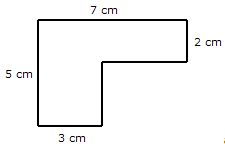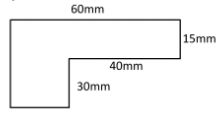# Perimeter of a piecewise rectangular figure

In this lesson, we find the perimeter of given piecewise rectangular figures.

We are given piecewise rectangular figures with some measurements while some other ones are missing. We first find these missing lengths. In these figures, it is assumed that all intersecting sides meet in right angles. It is noted that opposite sides of a rectangle are parallel and equal in length while calculating the missing lengths. We also split the given figure into two rectangles for same purpose.

Once the missing lengths are found, adding up the length along the outer boundary of the given figure gives the perimeter of the given figure.

Find the perimeter of the following figure. Notice that some length is not given. Assume that all intersecting sides meet at right angles.### Solution

Step 1:

Missing lengths 7 – 3 = 4 cm; 5 – 2 = 3 cm

Step 2:

Perimeter of given figure = 5 + 7 + 2 + 4 + 3 + 3 = 24 cm

Find the perimeter of the following figure. Notice that some length is not given. Assume that all intersecting sides meet at right angles.### Solution

Step 1:

Missing lengths 60 – 40 = 20 mm; 30 + 15 = 45 mm

Step 2:

Perimeter of given figure = 45 + 60 + 15 + 40 + 30 + 20 = 210 mm

Opposite sides of rectangles are of same length.

Now we can find the perimeter of the figure by adding all lengths.